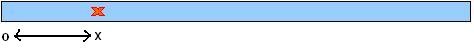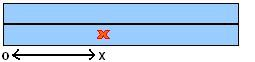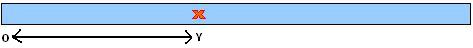# Stretching Fractions: A Discussion

##### Age 14 to 18

Published 2011 Revised 2018

First, to get some idea of what's going on, we should experiment with different starting fractions. It might be sensible to start with $\frac{1}{2}$ and then try $\frac{1}{3}$, $\frac{1}{4}$, and so on... here's what we get when we iterate these fractions: $$\frac{1}{2} \mapsto 1 \mapsto 0 \mapsto 0 \mapsto 0 \mapsto \cdots$$ $$\frac{1}{3} \mapsto \frac{2}{3} \mapsto\frac{2}{3} \mapsto\frac{2}{3} \mapsto \cdots$$ $$\frac{1}{4}\mapsto \frac{1}{2} \mapsto 1\mapsto 0 \mapsto 0 \mapsto 0 \mapsto \cdots$$ $$\frac{1}{5} \mapsto \frac{2}{5} \mapsto \frac{4}{5} \mapsto \frac{2}{5} \mapsto \frac{4}{5} \mapsto \cdots$$ $$\frac{1}{6} \mapsto \frac{1}{3} \mapsto \frac{2}{3} \mapsto \frac{2}{3} \mapsto \cdots$$ We notice that $\frac{1}{2}$ and $\frac{1}{4}$ both go to 0, which will then loop to itself for ever. In general which fractions will eventually go to 0?

The other fractions all end up in a different kind of loop. Lets try a more complex fraction: $$\frac{3}{14} \mapsto \frac{3}{7} \mapsto \frac{6}{7} \mapsto \frac{2}{7} \mapsto \frac{4}{7} \mapsto \frac{6}{7} \mapsto \cdots$$ Can you see that here the sequence goes back to $\frac{6}{7}$, and will continue going round in a loop of three fractions - $\frac{6}{7} \mapsto \frac{2}{7} \mapsto \frac{4}{7} \mapsto \frac{6}{7}$? Now for a bigger fraction: $$\frac{1}{120} \mapsto \frac{1}{60} \mapsto \frac{1}{30} \mapsto \frac{1}{15} \mapsto \frac{2}{15} \mapsto \frac{4}{15} \mapsto \frac{8}{15} \mapsto \frac{14}{15} \mapsto \frac{2}{15} \mapsto \cdots$$ Here the same kind of thing happens. We eventually track onto a loop beginning with $\frac{2}{15}$, which has four members: $\frac{2}{15} \mapsto \frac{4}{15} \mapsto \frac{8}{15} \mapsto \frac{14}{15} \mapsto \frac{2}{15}$.

You might have already asked yourself the following: Will all sequences end up in a loop no matter what our starting fraction is? It has been relatively easy to show that this is the case for our examples. But what about $\frac{240}{7436429}$, for instance? Can we find a clever argument to show that our conjecture is true in general , so that we can say that $\frac{240}{7436429}$ will track onto a loop without having to work it out?

What do we need in order for our sequence to contain a loop? Notice that in each of our examples above we know that there is a loop because some fraction occurs twice in the sequence. For example, we've worked out that if we start at $\frac{3}{14}$, then we get $\frac{6}{7}$ once, and then after three more iterations we get it again, and so after three more it'll appear again, and the sequence will repeat in groups of three forever. So given any sequence, if some fraction appears twice, then we know we must have a loop, and that this fraction will in fact occur infinitely many times. Once we've convinced ourselves that this is true, then proceeding with our argument is easier, since instead of finding loops explicitly, all we need to show is that for any sequence, there is a fraction that appears twice.

The rule we have been given for going from one fraction to the next is easy enough to explain, but a bit vague for a proper mathematical argument. Our first step is to translate the 'folding and stretching' rule into algebra. If we are given a fraction $\frac{p}{q}$ where $p$ and $q$ are whole numbers, what is the next fraction in terms of $p$ and $q$?

Suppose we mark our blu-tack at $X$. Then $\frac{p}{q}=\frac{OX}{1}=OX$.Let $\frac{p}{q}\leq \frac{1}{2}$. We fold (our mark does not move) and stretch, and our new fraction is $\frac{OY}{1}$.The important fact is that stretching preserves ratio, so $$\frac{OY}{1}=\frac{OX}{\frac{1}{2}}=2OX=\frac{2p}{q}$$ In other words, folding and stretching takes $\frac{p}{q}$ to $\frac{2p}{q}$.

If $\frac{p}{q}> \frac{1}{2}$ i.e. the mark is placed above the half way point, then folding moves the mark, taking $\frac{p}{q}$ to $1-\frac{p}{q}$ (can you see this?). Finally, stretching gives us $$2(1-\frac{p}{q}) =\frac{2(q-p)}{q}$$ Thus we have described the iteration process algebraically, breaking it down into the two seperate cases. Notice that the denominator of the second fraction is the same as that of our initial fraction, $q$, and if we iterate again, the denominator of our third fraction will be the same as that of our second, and so on... which means that every fraction in our sequence will have denominator $q$ (of course, we may be able to reduce the iterated fractions, but for the purpose of our argument we always leave the fraction as it is - you'll see why).

Now to the crux of the argument. We know that all fractions in the sequence starting at $\frac{p}{q}$ are between 0 and 1 and have denominator $q$, so any fraction must be one of $q+1$ possibilities: $$\frac{0}{q}, \frac{1}{q}, \frac{2}{q}, \cdots , \frac{q-1}{q}, \frac{q}{q}$$ This means that amongthe first $q+2$ fractions of the sequence, or any collection of $q+2$ fractions from the sequence, at least two must be the same, and we know that this means there must be a loop. So we're done - choose any starting fraction and our sequence will always track into a loop!

We have not discussed any interesting properties the loops might have. Investigate further. Given a number $n$, can we find a fraction that tracks onto a loop with $n$ elements? Experiment with particular values. Do all fractions with the same denominator track onto the same loop? If not, is there anything that the different loops share in common? Can you find a fraction with a very large denominator which tracks onto a relatively small loop?

We now look in another direction. What if we place a mark on the blue-tac that is not an exact fraction of its length, but such that the length $OX$ is an irrational number? This might seem like a strange thing to do, but in some sense there are many more irrational numbers than rational numbers,and if we mark the blue-tac at random, what is to say that our length $OX$ will be an exact fraction? So it's reasonable to investigate what happens if we apply the stretching and bending process to an irrational number. Will we track onto a loop?

Those of you who have dealt with irrational numbers before might guess that the answer to this question is no. Your intuition would be correct, and we go about proving this...

We show that the sequence generated by an irrational number will contain only irrational numbers, and then show that these irrational numbers will all be different.

If we could use our algebraic form of the iteration rule to write all terms in the sequence as a function of our starting fraction then we'd be in a strong position, but problems arise in our case: our formula is different for values either side of $\frac{1}{2}$, so to write an explicit expression for the $n$th term would involve knowing at each stage whether our mark is greater of less than $\frac{1}{2}$, and things soon start to get difficult.

However, there is a way around this: we might not be able to work out an exact expression for each term exactly, but we can show what form this expression will take, and that is enough for our purposes.

If we start our sequence at some number $x$ (between $0$ and $1$), then any term in the sequence will be of the form $ax+b$, where $a$ and $b$ are integers and $a\ne 0$ or $1$.

This is certainly true for the second term of the sequence, which is either $2x$ or $2(1-x)=2-2x$, and if it is true for the $n$th term, which is $a_nx+b_n$, say, then the $n+1$th term will be either $2a_nx+2b_n$ or $-2a_nx-2b_n+2$. So an induction argument proves this statement.

Now, if $x$ is irrational and some term $ax+b$ in its sequence is rational, then for some integers $p$ and $q$ we can write it as $$ax+b=\frac{p}{q}$$ $$\Rightarrow x=\frac{p-qb}{aq}$$ Since $a\ne 0$ this is a valid expression whichshows that $x$ is rational, which is nonsense. So $ax+b$ cannot be rational, and so no term in the sequence can be rational.

Now, for two members of the sequence to be the same i.e. there is some irrational number $y$ which goes back to $y$ after a certain number of iterations, then for some other token integers $a$ and $b$, $$ay+b=y$$ $$\Rightarrow y=\frac{b}{1-a}$$ $a\ne 1$ so this is valid, therefore y is rational - CONTRADICTION.

Therefore it is impossible to get a loop in the sequence, since for this to happen two terms must be the same.